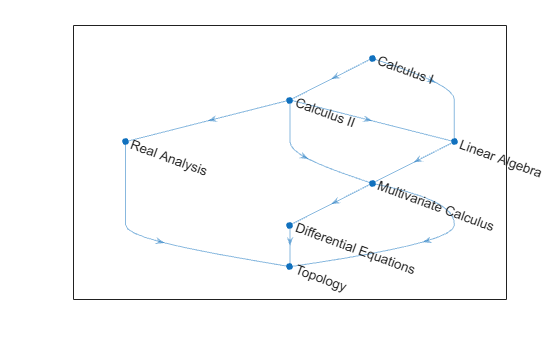Main Content

# toposort

Topological order of directed acyclic graph

## Syntax

``n = toposort(G)``
``n = toposort(G,'Order',algorithm)``
``````[n,H] = toposort(___)``````

## Description

example

````n = toposort(G)` returns the topological order of the nodes in `G` such that `i < j` for every edge `(n(i),n(j))` in `G`. The directed graph `G` cannot have any cycles.```

example

````n = toposort(G,'Order',algorithm)` specifies the ordering algorithm. For example, `toposort(G,'Order','stable')` uses a stable ordering algorithm based on the lexicographical order of the nodes.```

example

``````[n,H] = toposort(___)``` additionally returns directed graph `H` whose nodes are in the given topological order. You can use any of the input argument combinations in previous syntaxes.```

## Examples

collapse all

Create and plot a graph that represents a progression of university-level Mathematics courses. An edge between two courses signifies a course requirement.

```A = [0 1 1 0 0 0 0 0 0 0 1 0 0 0 0 1 0 1 0 0 1 0 0 0 0 1 1 0 0 0 0 0 0 0 0 0 0 0 0 1 0 0 0 0 0 0 1 0 0]; names = {'Calculus I','Linear Algebra','Calculus II', ... 'Multivariate Calculus','Topology', ... 'Differential Equations','Real Analysis'}; G = digraph(A,names); plot(G)```Find the topological sorting of the courses to determine the proper order in which they should be completed.

`N = toposort(G)`
```N = 1×7 1 3 7 2 4 6 5 ```
`G.Nodes.Name(N,:)`
```ans = 7x1 cell {'Calculus I' } {'Calculus II' } {'Real Analysis' } {'Linear Algebra' } {'Multivariate Calculus' } {'Differential Equations'} {'Topology' } ```

Create a directed graph using a logical adjacency matrix, and then plot the graph.

```rng default; A = tril(sprand(10, 10, 0.3), -1)~=0; G = digraph(A); [~,G] = toposort(G); plot(G)```Find the topological sorting of the graph nodes. Even though `G` is already in topological order, `(1 2 3 4 5 6 7 8 9 10)`, `toposort` reorders the nodes.

`toposort(G)`
```ans = 1×10 2 1 4 10 9 8 5 7 3 6 ```

Specify `Order` as `'stable'` to use the stable ordering algorithm, so that the sort orders the nodes with smaller indices first. Stable sort does not rearrange `G` if it is already in topological order.

`toposort(G,'Order','stable')`
```ans = 1×10 1 2 3 4 5 6 7 8 9 10 ```

## Input Arguments

collapse all

Input graph, specified as a `digraph` object. `G` must be a directed acyclic graph. Use `isdag` to confirm that `G` does not contain cycles.

Use `digraph` to create a directed graph.

Example: `G = digraph([1 2],[2 3])`

Ordering algorithm, specified as `'fast'` or `'stable'`:

 `'fast'` (default) Based on a depth-first search. A node is added to the beginning of the list after considering all of its descendants.If `G` is already in topological order, this method might still reorder the nodes. `'stable'` Based on lexicographical order. `n(1)` is the node with smallest index, `n(2)` the next smallest given `n(1)`, and so on. If `G` is in topological order then `H` is unchanged and `n` is `1:numnodes(G)`.

Example: ```[n,H] = toposort(G,'Order','stable')```

## Output Arguments

collapse all

Node indices, returned as a row vector.

Topologically sorted graph, returned as a `digraph` object. `H` is the same graph as `G`, but has the nodes reordered according to `n`.

## More About

collapse all

### Topological Order

The topological ordering of a directed graph is an ordering of the nodes in the graph such that each node appears before its successors (descendants).

Consider a directed graph whose nodes represent tasks and whose edges represent dependencies that certain tasks must be completed before others. For such a graph, the topological sorting of the graph nodes produces a valid sequence in which the tasks could be performed.

## See Also

Introduced in R2015b

Download ebook